# JavaScript基础

## 2.基本语法

### 2.1内部js

<script>
</script>


### 2.1外部js

<head>
<meta charset="UTF-8">
<title>Title</title>

<script src="js1.js"></script>

<!--<script>
</script>-->


### 2.3注释

1.单行注释

//单行注释

2.多行注释

/*  多行*/


### 2.4数据类型

1.（原始）基本数据类型

a、number 整数、小数、NaN(not a number 一个不是数字的数字类型)

console.log(isNaN(12));//false


isNaN 判断是否为非数字 结果为false 说明不是非数字 即数字

b、string

alert('放，啦【积分是丰富\n的非暗示领导立刻拉多少；\n"阿萨德d"sF非空FE');


②字符串拼接

var as='abc de';
console.log((as.length));
var aw=as+123;
console.log(aw);


console.log('10'+10);//1010


c、boolean

d、null

e、undefined 未定义

2.引用数据类型

##### 2.3.1数据类型转换

1.把数字转为字符串

• toString( )
• String(变量)
• 拼接 变量+’ ’

2.转化为数字类型

paserInt( ) 注意：取整了

paserFloat( ) 浮点数

Number（ ）强转

### 2.4变量 *

js不同于Java

Java是强类型语言 在开辟变量存储空间的同时，定义了空间将来存储的数据的数据类型，只能存储固定类型的数据

var a=10;


br换行

var a=1;
var b=1.2;
var c=NaN;

document.write(a+'<br>');
document.write(b+'<br>');
document.write(c);

var a1='abc';
document.write(a1);

var a01=true;
document.write(a01+'<br>');

var b1=null;
var b01=undefined;
document.write(b1+'<br>');
document.write(b01+'<br>');


ocument.write(a+'---'+typeof(a)+'<br>');
document.write(b+'<br>');
document.write(c+'<br>');

var a1='abc';
document.write(a1+'---'+typeof(a1)+'<br>');

var a01=true;
document.write(a01+'---'+typeof(a01)+'<br>');

var b1=null;
var b01=undefined;
document.write(b1+'---'+typeof(b1)+'<br>');
document.write(b01+'---'+typeof(b01)+'<br>');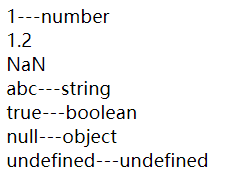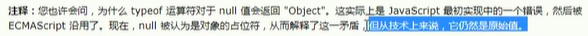##### 案例：

1.输入用户名并弹出显示用户名

var myname=prompt('请输入你的用户名');


2.更新变量

var myname='smy';
console.log(myname);


myname='hhh';
console.log(myname);


3.声明多个变量

var age=18,


3.1只声明不赋值

var sex;
console.log(sex);//undefined


3.2不声明不赋值会报错

3.3不声明直接赋值

aa=10;
console.log(aa);//10


### 2.5运算符

1.一元运算符：只有一个运算数的运算符

string按照字面值转换，如果不是数字，则转为NaN

boolean转，true为1 false为0

var a=1;
var b= -a;
document.write(b+'<br>');
document.write(a);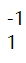var a=+'123';
var b=1+a;
document.write(b+'<br>');
document.write(a);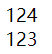var a=+'abc';
var b=1+a;
document.write(b+'<br>');
document.write(a);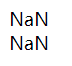2.算数运算符

3.赋值运算符

= += -= 。。。。

4.比较运算符

< > >= <= == ===(全等于)

document.write('abc'>'acd'+'<br>')


//false

c比b大 d比c大

document.write(('123'>1)+'<br>')


true

5.逻辑运算符

&& || ！

6.三元运算符

？ ：

## 3.数组

### 3.1创建数组

1.new

var 数组名=new Array();

2.利用数组字面量( [ ] )创建

var 数组名=[ 1,2,23];

## 4.函数

*注意：在js中 如果实参的个数多于形参个数 会取到形参个数

​ 如果小于， 因为形参看作是不用声明的变量 ，此时形参没有接受值 定义为undefined 数字+undefined=NaN

### 4.1return

return是把结果返回给调用者 且终止函数

function g(num1,num2){
return [num1,num2];
}
console.log(g(1,2));


### 4.2return，break，continue的区别

return：退出循环，返回值，同时结束当前函数体代码

break：结束当前循环体

continue:跳出本次循环，继续执行下次循环

### 4.3arguments

arguments是函数的一个内置对象，所有函数都内置了一个arguments对象，arguments对象中存储了传递的所有实参

#### 4.3.1使用

function f() {
console.log(arguments);
}
f(1,2,3);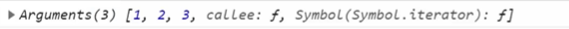1.具有数组的length属性

2.按照索引方式进行存储

3.没有真正数组的一些方法 如pop() push()等

function getMax() {
var max=arguments;
for (var i=1;i<arguments.length;i++){
if (arguments[i]>max){
max=arguments[i];
}
}
return max;
}
console.log(getMax(1,34,23,45));


### 4.4函数的声明

1.利用函数关键字自定义函数（命名函数

function f(){

}

2.函数表达式（匿名函数

var f=function(){

};

js内可以函数内定义函数

## 5.预解析*

1.js引擎运行js分为：预解析，代码执行

​ 预解析 js引擎会把js里面所有的var 和function 提升到当前作用域的最前面

​ 代码执行：从上往下

2.预解析分为：变量预解析（变量提升）和函数与解析（函数提升）

console.log(num);//undefined
var num=10;


f();
function f(){
console.log(22);
}//相当于先声明了把这整个函数提到前边去 之后在调用


### 案例：

var num=10;
f();  //undefined
function f() {

console.log(num);
var num=20;
}


var num;
function f() {

var num;
console.log(num);
num=20;
}
num=10;
f();


### 经典案例：

f();
console.log(c);
console.log(b);
console.log(a);
function f() {
var a=b=c=9;
console.log(a);
console.log(b);
console.log(c);

}


var a=b=c=9; 相当于： var a=9; b=9; c=9; b，c直接复制没声明 当全局变量看

function f() {
var a;
a=b=c=9;
console.log(a);
console.log(b);
console.log(c);
}
f();
console.log(c);
console.log(b);
console.log(a);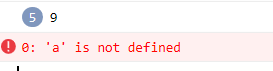f();
console.log(c);
console.log(b);
console.log(a);
function f() {
var a,b,c=9;
console.log(a);
console.log(b);
console.log(c);

}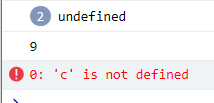## 6.对象

### 字面量

var obj={};

var obj={
name:'my',
age:18,
sex:'man',
sayHi:function () {
console.log('hi!')
}

}


a、里面的属性或方法采用键值对形式 键 属性名 ： 值 属性值

b、用逗号, 隔开

c、方法冒号后边跟的是一个匿名函数

a、调用对象的属性，采用 对象名.属性名

console.log(obj.age);


b、调用属性方法二 对象名[‘属性名’]

console.log(obj['age']);



c、调用对象的方法 对象名.方法名( ) 不要忘记( )

obj.sayHi();


2.new

#### 补充：变量、属性、函数、方法的区别?

1.变量和属性 都是用来存数据的

var num=18;
var obj={
age:18
}
console.log(num);
console.log(obj.age);


2.函数和方法 都是用来实现某种功能 做某件事

var obj={
age:18,
hh:function () {
console.log('fd');
}
}
function f(){

}


### 6.2 new Object

var obj=new Object( );//创建了一个空的对象

var obj=new Object( );
obj.name='smy';
obj.age=18;
obj.sex='man';
obj.sayHi=function () {
console.log('hi');
}
console.log(obj.age);
obj.sayHi();


1.利用= 等号赋值的方法 添加对象的属性和方法

2.每个属性和方法之间 用 分号; 结束

### 6.3利用构造函数

#### 6.3.1语法格式：

/*function 构造函数名() {
this.属性=值;
this.方法=function () {}
}
new 构造函数名();*/

function Stu(name,age,sex) {
this.name=name;
this.age=age;
this.sex=sex;
this.job=function (job) {
console.log(job);
}
}
var xm= new Stu('小明',18,'男');//调用函数返回的是一个对象
console.log(xm.age);
xm.job('code');
var nana=new Stu('nana',17,'女');
console.log(nana.sex);


1.构造函数名字首字母要大写

2.构造函数不需要return就可以返回结果

3.调用构造函数 用new

4.只要new Stu( ) 调用函数就创建一个对象

5.属性和方法前面必须添加this

#### 6.3.2构造函数和对象的区别？

1.构造函数 泛指某一大类 抽象了对象的公共部分

​ 类似于Java里的class类 如学生

2.对象是一个具体事物 如小明同学

3.利用构造函数创建对象的过程也称为对象的实例化

#### 6.3.3new关键字执行过程

1.new 构造函数 在内存中创建一个空的对象

2.this就会指向刚才创建的空对象

3.执行构造函数里面的代码，给这个空对象添加属性和方法

4.返回这个对象（所以不需要return）

### 6.4遍历对象for…in

for(变量 in 对象){

}

var obj={
name:'xm',
age:18,
hh:function () {
console.log('fd');
}
}
for(var k in obj){
console.log(k);//k 变量 输出 得到的是属性名
console.log(obj[k]);//得到的是属性值
}


## 7.内置对象

### 7.1概述

js中的对象分为3种：自定义对象、内置对象、浏览器对象

### 7.2查文档

MDN/W3C

https://developer.mozilla.org/zh-CN/

### 7.3Math

1.Math数学对象 不是一个构造函数，所以不需要用new来调用，而是直接使用里面的属性和方法即可

console.log(Math.PI);
console.log(Math.max(23,1,34));


var myMath={
PI:3.141592653,
max:function () {
var max=arguments;
for (var i=1;i<arguments.length;i++){
if(arguments[i]>max){
max=arguments[i];
}
}
return max;
},
min:function () {
var min=arguments;
for (var i=1;i<arguments.length;i++){
if(arguments[i]<min){
min=arguments[i];
}
}
return min;
}
}
console.log(myMath.PI);
console.log(myMath.max(2,4,5));
console.log(myMath.min(3,1,0));


2.绝对值abs

console.log(Math.abs(-1));//1
console.log(Math.abs('-1'));// 1 隐式转换
console.log(Math.abs('pink'));//NaN


3.取整

console.log(Math.floor(1.1));//1
console.log(Math.floor(1.9));//1 向下取整

console.log(Math.ceil(1.1));//2 向上取整
console.log(Math.ceil(1.9));//2

//四舍五入
console.log(Math.round(1.1));//1
console.log(Math.round(1.4));//1
console.log(Math.round(1.5));//2
//注意
console.log(Math.round(-1.1));//-1
console.log(Math.round(-1.4));//-1
console.log(Math.round(-1.5));//结果是-1  其他数字都是四舍五入 但5特殊，往大了取
console.log(Math.round(-1.6));//-2


4.随机数

console.log(Math.random());//[0,1) 小数
console.log(Math.random()*(10-0)+0);//[0,10)的数 小数或整数

// Math.floor(Math.random()*(max-min)+min)取整
//  Math.floor(Math.random()*(max-min+1)+min)左闭右闭

//封装成函数
function getRandom(min,max) {
return Math.floor(Math.random()*(max-min+1)+min);

}
console.log(getRandom(1,10));
var stu=['xm','xa','aa'];
console.log(stu[getRandom(0,stu.length-1)]);


##### 随机数案例：
var min=prompt('请输入范围最小值');
var max=prompt('请输入范围最大值');
function getRandom(min,max) {
return Math.floor(Math.random()*(max-min+1)+min);

}

var result=getRandom(min,max);
var num=prompt('请输入猜测的数');
pd(num);
function pd(num){
if (num==result){
console.log('恭喜你猜对了！答案是：'+result);

}else{
if(num>result){
var num1= prompt('大了,请重新输入');
pd(num1);

}else{
var num2=prompt('小了，请重新输入');
pd(num2);
}
}
}


### 7.4Date

Date( )日期对象 是一个构造函数 必须实例化

1.使用Date 如果没有参数，返回当前系统当前时间

var date =new Date();
console.log(date);


2.参数常用的写法 数字型 2020,06,29 或者是字符串型 ‘2020-6-29 8:8:8’

var date1=new Date(2020,6,29);
console.log(date1);//返回的是七月
//Wed Jul 29 2020 00:00:00 GMT+0800 (中国标准时间)

console.log(date.getFullYear());//返回当前的年
console.log(date.getMonth()+1);//返回的月份小一个月 所以记得月份+1
console.log(date.getDate());
console.log(date.getDay());//0-6 周日为0



var date =new Date();
var year=date.getFullYear();
var month=date.getMonth()+1;
var dates=date.getDate();
var arr=['周日','周一','周二','周三','周四','周五','周六'];
var day=date.getDay();
console.log(year+'年'+month+'月'+dates+'日'+arr[day]);

//2020年6月29日周一


function getTime(){
var time=new Date();
var hour=time.getHours();
hour= hour<10?'0'+hour:hour;
var mi=time.getMinutes();
mi= mi<10?'0'+mi:mi;
var s=time.getSeconds();
s =s< 10 ? '0' + s : s ;
return hour+':'+mi+':'+s;
}

console.log(getTime());//17:34:02


Date对象基于1970年1月1号

​ 方法a：通过valueOf() getTime()

var date=new Date();
console.log(date.valueOf());//距1970.1.1的毫秒数
console.log(date.getTime());//同上


​ 方法b：简单写法（最常用写法）

var date1= + new Date();//+ new Date() 返回的是总的毫秒数
console.log(date1);


​ 方法c：最最简单写法（h5新增）所以需要考虑兼容性

console.log(Date.now());


#### 倒计时案例***

1、核心算法：输入的时间减去现在的时间=倒计时

​ 但是！不能用时分秒相减 比如02-20=-18

2、用时间戳来做，用户输入时间总的毫秒数，得到的就是剩余时间的毫秒数

3、把剩余时间总的毫秒数转换为天、时、分、秒（时间戳转换为时分秒）

function countDown(time) {//countDown 倒计时 传入的是用户输入时间
var nowTime=+new Date();//返回的是当前时间总的毫秒数
var inputTime= + new Date(time);//返回的是用户输入时间总的毫秒数
var times= (inputTime-nowTime)/1000;//time是剩余时间总的毫秒数  /1000变成秒

var d=parseInt(times/60/60/24);//天数
d =d< 10 ? '0' + d : d ;
var h=parseInt(times/60/60%24);//时
h =h< 10 ? '0' + h : h;
var m=parseInt(times/60%60);//分
m =m< 10 ? '0' + m : m ;
var s=parseInt(times%60);//当前的秒
s =s< 10 ? '0' + s : s ;

return d+'天'+h+'时'+m+'分'+s+'秒';
}
var date=new Date();
console.log('现在是'+date);

console.log('距离目标时间还有：'+countDown('2020-6-29 23:00:00'));
//
/*

js1.js (294,1)



### 7.5数组对象Array

1.利用数组字面量

var arr=[1,2,3];

console.log(arr);

2.利用new Array()

var arr1=new Array();//创建了一个空数组

var arr1=new Array(2);//这个2表示数组的长度为 2 里面有2个空的数组元素；

var arr1=new Array(2,3);//等价于[2,3]这样写表示里面有2数组元素 是2和3

#### 7.5.1检测是否为数组：

1.instanceof 运算符

//翻转数组
function reverse(arr) {
if(arr instanceof Array){
var newArr=[];
for (var i=arr.length-1;i>=0;i++){
newArr[newArr.length]=arr[i];

}
return newArr;

}else{
return '这个参数要求必须是数组格式[1,2,3]';
}
}

console.log(reverse([1,2,3]));
console.log(reverse(1,2,3));
//3,2,1
//这个参数要求必须是数组格式[1,2,3]


2.Array.isArray() H5新增 ie9以上版本支持

 var arr=[];
var obj={};
console.log(arr instanceof Array);
console.log(obj instanceof Array);

console.log(Array.isArray(arr))
console.log(Array.isArray(obj));


#### 7.5.2添加删除数组元素的方法

1.push( ) 在数组末尾 添加一个或多个数组元素 push推

var arr=[1,2,3];
arr.push(4,'xm');
console.log(arr);//[object Array]: [1, 2, 3, 4, "xm"]

console.log(arr.push(4,'xm'));//5 返回的是新数组长度


2.unshift（） 在数组开头 添加一个或多个数组元素

arr.unshift('red');
console.log(arr);
console.log(arr.length);
//[object Array]: ["red", 1, 2, 3, 4, "xm"]  6

console.log(arr.unshift('red'));//6 返回的是新数组长度


3.pop() 删除数组最后一个元素

arr.pop();
console.log(arr);//[object Array]: ["red", 1, 2, 3, 4]

console.log(arr.pop());//xm 返回的是删除的元素


4.shift() 删除第一个元素

arr.shift();
console.log(arr);//[object Array]: [1, 2, 3, 4]

console.log(arr.shift());//red


var arr=[1500,2000,2000,1800,2000,2100,2000];
var newArr=[];
for (var i=0;i<arr.length;i++){
if (arr[i]!=2000){
newArr.push(arr[i]);
}
}
console.log(newArr);//[object Array]: [1500, 1800, 2100]


#### 7.5.3数组排序

1.翻转数组 recerse()

var arr=[1,2,4];
arr.reverse();
console.log(arr);//[object Array]: [4, 2, 1]


2.数组排序（冒泡排序） **

var arr0=[2,1,7,4];
arr0.sort();
console.log(arr0);//[object Array]: [1, 2, 4, 7]

var arr=[1,2,4,23,21,6];
arr.sort();
console.log(arr);//[object Array]: [1, 2, 21, 23, 4, 6]


arr.sort(function (a,b) {
return a-b;//升序
});
console.log(arr);//[object Array]: [1, 2, 4, 6, 21, 23]

arr.sort(function (a,b) {
return b-a;//降序
});
console.log(arr);//[object Array]: [23, 21, 6, 4, 2, 1]


#### 7.5.4数组索引

1.indeOf()

var arr=[1,'red',23,'ww','red'];
var n=arr.indexOf('red');
console.log(n);// 1  只返回第一个满足条件的索引号
console.log(arr.indexOf('blue'));//-1 表示没有

console.log(arr.lastIndexOf('red'));//4 从后面开始查找 但是索引号不会变
console.log(arr.lastIndexOf('blue'));//-1


#### 利用indexOf（）的数组去重案例***

var arr=[1,2,3,1,4,3,5,6,4,1];
function unique(arr) {
var newArr=[];
for (var i=0;i<arr.length;i++){
if(newArr.indexOf(arr[i]) === -1){//新数组里没有这个元素
newArr.push(arr[i]);//就添加进去
}
}
return newArr;
}

console.log(unique(arr));//[object Array]: [1, 2, 3, 4, 5, 6]


#### 7.5.5数组转化为字符串

1.toString()

var arr=[1,2,3];
console.log(arr.toString());//1,2,3


2.join(分隔符)

console.log(arr.join());//1,2,3
console.log(arr.join('&'));//1&2&3


3.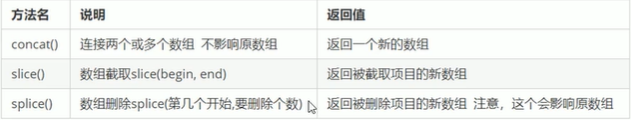### 7.6字符串对象

var str='jenny';
console.log(str.length);//5


#### 7.6.1基本包装类型：就是把简单数据类型 包装成为了 复杂数据类型

String Number Boolean 都有

1.把简单数据类型包装为复杂数据类型

var temp=new String('jenny');


2.把临时变量的值 给 str

str =temp;


3.销毁这个临时变量

temp=null;


#### 7.6.2字符串的不可变性

var str='andy';
console.log(str);//andy
str='red';
console.log(str)//red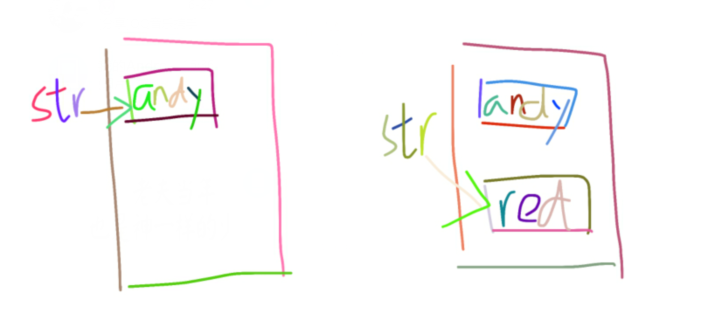var str='';
for (var i=1;i<=100;i++){
str+=i;
}
console.log(str);//如果数再大就会卡会等好久


#### 7.6.3根据字符返回位置

1.indeOf()

var str='改革春风吹满地,春天来了';
console.log(str.indexOf('春'));//2

console.log(str.indexOf('春',));//8


【3】从索引为3处开始查找

var str='sjfosdbfoooscsop';
var index=str.indexOf('o');
var num=0;
while (index !== -1){
console.log(index);//先输出 再找下一轮结果
index=str.indexOf('o',index+1);
num+=1;
}
console.log(num);

var arr=['red','blue','red','red','pink'];
var index=arr.indexOf('red');
var num=0;

while (index !== -1){
console.log(index);//先输出 再找下一轮结果
index=arr.indexOf('red' ,index+1);
num+=1;
}
console.log(num);


#### 7.6.4根据位置返回字符***

1.charAt（index）根据位置返回字符

var str='andy';
console.log(str.charAt(3));//y
//遍历所有字符
for (var i=0;i<str.length;i++){
console.log(str.charAt(i));
}

###### 2**.charCodeAt(index) 返回相应索引号的字符ASCII值

3.str[index] h5新增

console.log(str);//a


var str='sjfosdbfoooscsop';
var num={};
for (var i=0;i<str.length;i++){
var chars=str.charAt(i);
if (num[chars]){
num[chars]++;

}else{
num[chars]=1;//得到的是属性值
}
}
console.log(num);
/*b: 1
c: 1
d: 1
f: 2
j: 1
o: 5
p: 1
s: 4*/
//遍历对象
var max=0;
var ch='';
for (var k in num){
if (num[k] >max){
max=num[k];
ch=k;
}
}
console.log(max);
console.log('最多的字符是：'+ch);
/*
5



#### 7.6.5字符串的操作***

1.拼接 conact（‘str1’,‘str2’,‘str3’）

var str='andy';
console.log(str.concat('red'));//andyred


2.截取 substr(‘截取的起始位置’,‘截取几个字符’)

var str='改革春风吹满地';
console.log(str.substr(2,2));//春风


3.替换 replace(‘被替换的字符’,‘替换为的字符’)

var str='andy';
console.log(str.replace('a','b'));//bndy
var str='andyandy';
console.log(str.replace('a','b'));//bndyandy  只替换第一个字符


var str='sdadfuckfdsfuckssa';
while (str.indexOf('fuck')!==-1){
str=str.replace('fuck','****');
}


4.字符串转换为数组 split(‘分隔符’)

var str='red,pink,green';
console.log(str.split(','));//[object Array]: ["red", "pink", "green"]


5.转换大小写

var str='aBc';
console.log(str.toLowerCase());//abc
console.log(str.toUpperCase());//ABC


## 8.小结***：简单数据类型与复杂数据类型

### 8.1简单数据类型与复杂数据类型

1.值类型:在存储变量中存储的是值本身，因此叫做值类型

null特殊：

var timer=null;
console.log(typeof timer);//返回的是一个空的对象 object
//如果有个变量我们打算以后存储为对象，暂时没想好放啥，这时候就给null


2.引用类型：在存储变量中存储的仅仅是地址（引用），因此叫做引用数据类型

### 8.2堆和栈

1.栈（操作系统）：由操作系统自动分配释放存放函数的参数值、局部变量的值等。其操作方式类似于数据结构中的栈；

2.堆（操作系统）：；存储复杂类型（对象），一般由程序员分配释放，若程序员不释放，由垃圾回收机制回收。

js中并没有堆栈概念，通过堆栈来理解执行方式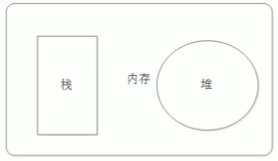### 8.4复杂类型的内存分配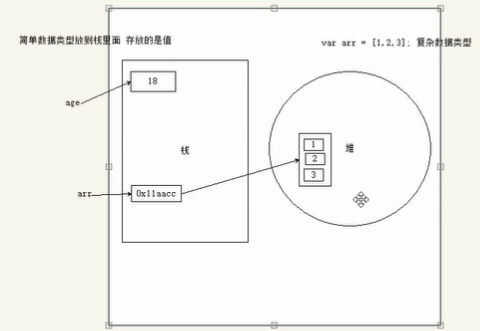### 8.5简单类型传参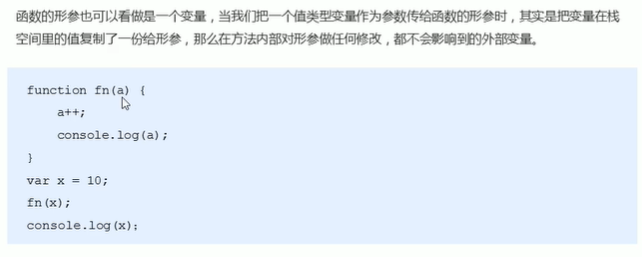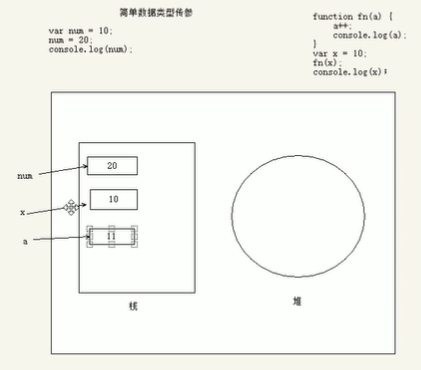### 8.6复杂类型传参

#### JavaScript基础入门到精通（网站开发就业技能）

09-0812-31

#### javascript零入门实战系列

09-26

12-181万+

11-29

03-21

06-11

08-14

#### JavaScript+jQuery+项目实战系列视频教程

10-27©️2020 CSDN 皮肤主题: 大白 设计师: CSDN官方博客点击重新获取扫码支付1.余额是钱包充值的虚拟货币，按照1:1的比例进行支付金额的抵扣。
2.余额无法直接购买下载，可以购买VIP、C币套餐、付费专栏及课程。余额充值